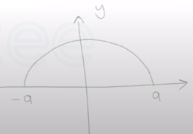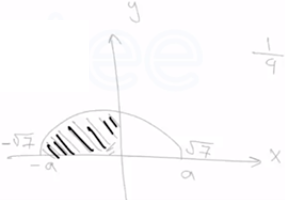Need Help?

Subscribe to Calculus A

###### \${selected_topic_name}
• Notes

Evaluate the following integral $\int_{-3}^{3} \sqrt{9-x^{2}} \mathrm{d} x$$\int_{-3}^{3} \sqrt{9-x^{2}} d x=\frac{1}{2} \pi a^{2}$

$a^{2}=(3)^{2}=9$

$\int_{-3}^{3} \sqrt{9-x^{2}} d x=\frac{1}{2} \pi(9)=\frac{9}{2} \pi$

Evaluate the following integral $\int_{-\sqrt{7}}^{0} \sqrt{7-x^{2}} \mathrm{d} x$$\int_{-\sqrt{7}}^{0} \sqrt{7-x^{2}} d x=\left(\frac{1}{2} \pi a^{2}\right) \frac{1}{2}$

$=\left(\frac{1}{2} \pi(\sqrt{7})^{2}\right) \frac{1}{2}$

$=\frac{1}{4} \pi 7=\frac{7}{4} \pi$

Use part 1 of the Fundamental Theorem of Calculus to find the derivative of the function $g(x)=\int_{0}^{x} \sqrt{t+t^{3}} d t$

$g(x)=\int_{0}^{x} \sqrt{t+t^{3}} d t=$

$g(x)=\sqrt{x+x^{3}}$